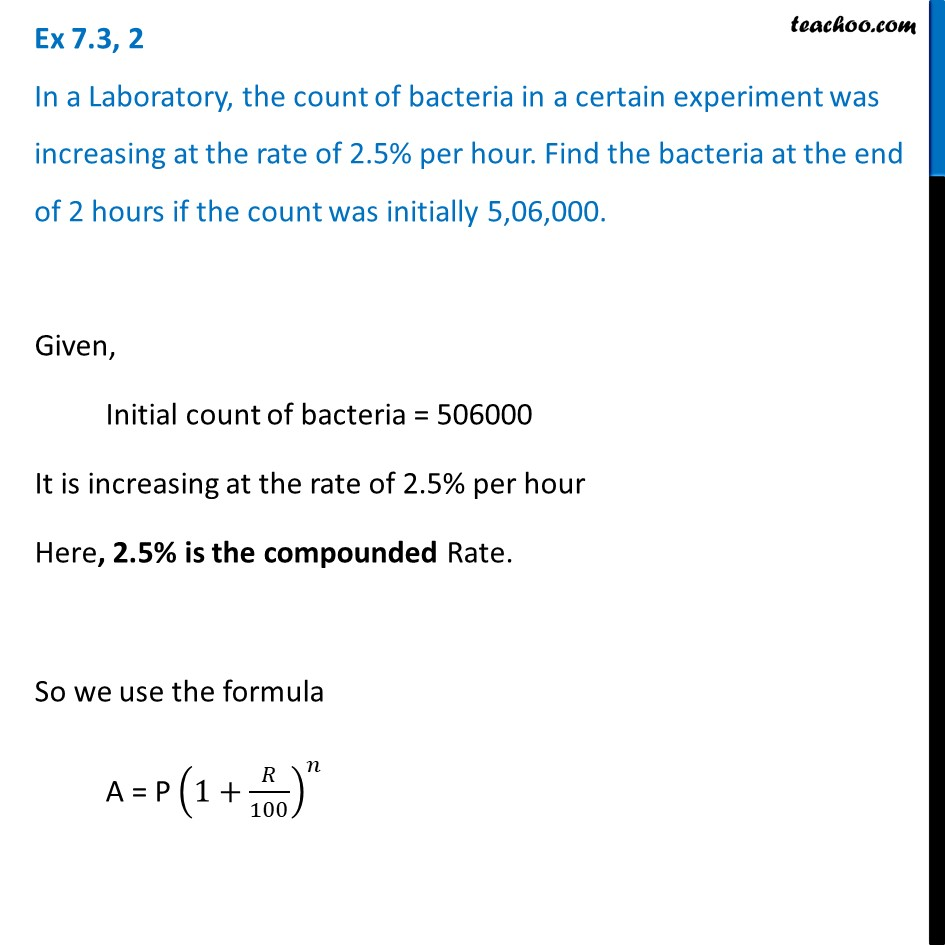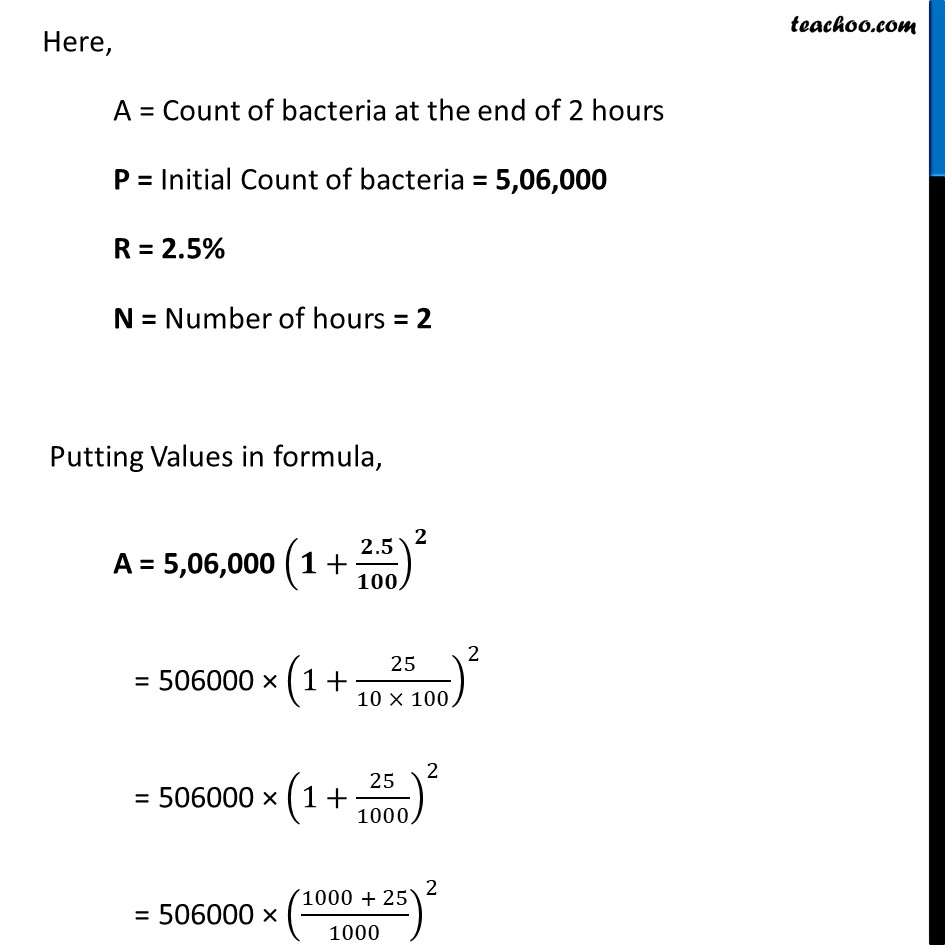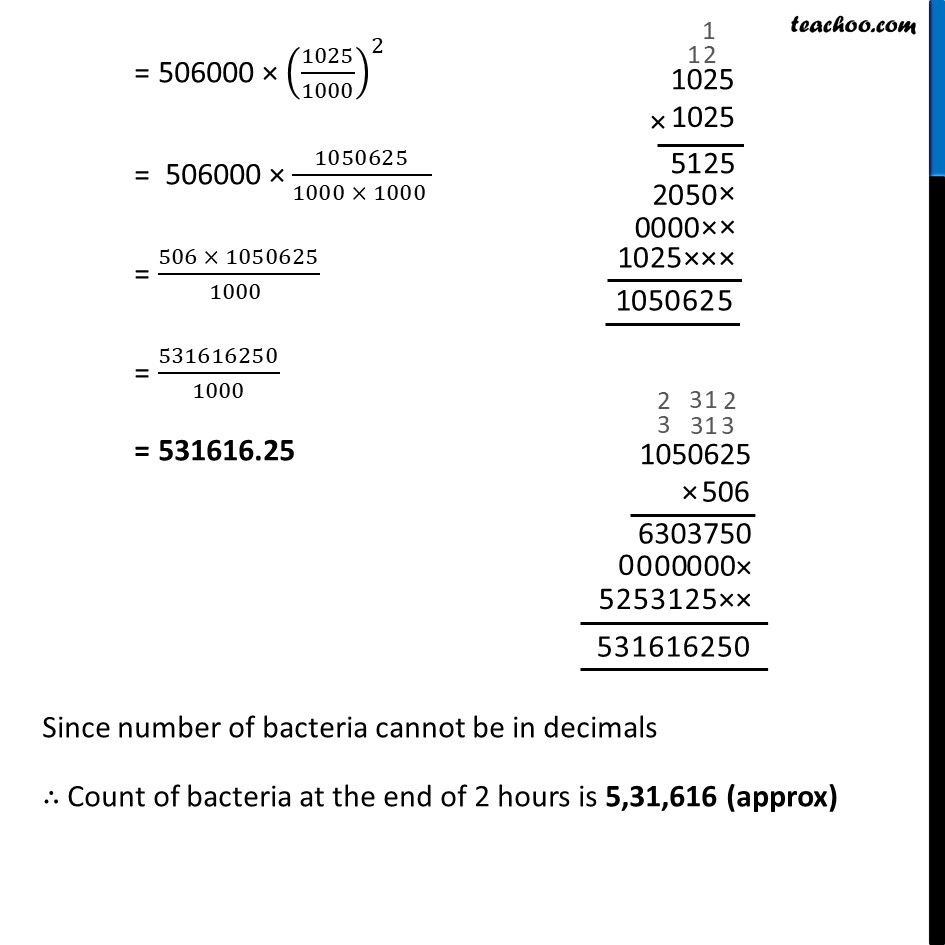Ex 7.3

Chapter 7 Class 8 Comparing Quantities
Serial order wiseLearn in your speed, with individual attention - Teachoo Maths 1-on-1 Class

### Transcript

Ex 7.3, 2 In a Laboratory, the count of bacteria in a certain experiment was increasing at the rate of 2.5% per hour. Find the bacteria at the end of 2 hours if the count was initially 5,06,000.Given, Initial count of bacteria = 506000 It is increasing at the rate of 2.5% per hour Here, 2.5% is the compounded Rate. So we use the formula A = P (1+𝑅/100)^𝑛 Here, A = Count of bacteria at the end of 2 hours P = Initial Count of bacteria = 5,06,000 R = 2.5% N = Number of hours = 2 Putting Values in formula, A = 5,06,000 (𝟏+(𝟐.𝟓)/𝟏𝟎𝟎)^𝟐 = 506000 × (1+25/(10 × 100))^2 = 506000 × (1+25/1000)^2 = 506000 × ((1000 + 25)/1000)^2 = 506000 × (1025/1000)^2 = 506000 × 1050625/(1000 × 1000 ) = (506 × 1050625)/(1000 ) = 531616250/1000 = 531616.25 Since number of bacteria cannot be in decimals ∴ Count of bacteria at the end of 2 hours is 5,31,616 (approx)